Students can Download Tamil Nadu 12th Chemistry Model Question Paper 1 English Medium Pdf, Tamil Nadu 12th Chemistry Model Question Papers helps you to revise the complete Tamilnadu State Board New Syllabus, helps students complete homework assignments and to score high marks in board exams.

## TN State Board 12th Chemistry Model Question Paper 1 English Medium

Time: 3 Hours
Maximum Marks: 70

Instructions:

1. The question paper comprises of four parts
2. You are to attempt all the parts An internal choice of questions is provided wherever applicable
3. All questions of Part I, II, III, and IV are to be attempted separately
4. Question numbers 1 to 15 in Part I are Multiple Choice Questions of one mark each. These are to be answered by choosing the most suitable answer from the given four alternatives and writing the option code and the corresponding answer
5. Question numbers 16 to 24 in Part II are two-mark questions. These are lo be answered in about one or two sentences
6. Question numbers 25 to 33 in Part III are three-marks questions. These are to be answered in about three to five short sentences
7. Question numbers 34 to 38 in Part IV are five-mark Questions These are to answered in detail. Draw diagrams wherever necessary

Part – 1

Answer all the questions. Choose the correct answer. [15 × 1 = 15]

Question 1.
Match items in column -I with the items of column – II and assign the correct code: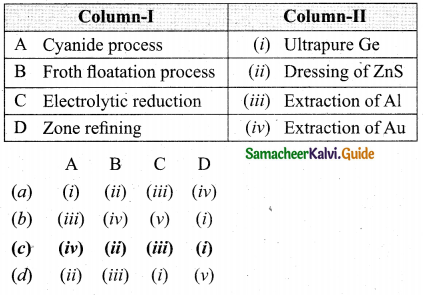(c) A – (iv), B – (ii), C – (iii), D – (i)

Question 2.
Which one of the following is double salt?
(a) Potash alum
(b) Potassium sulphate
(c) Aluminium Sulphate
(d) Ammonium sulphate
(a) Potash alum

Question 3.
Most easily liquefiable gas is ……………..
(a) Ar
(b) Ne
(c) He
(d) Kr
(c) HeQuestion 4.
Which metal is used in manufacturing artificial joints?
(a) Molybdenum
(b) Titanium
(c) Tungsten
(d) Iron
(b) Titanium

Question 5.
In which of the following coordination entities the magnitude of A will be maximum?
(a) [CO(CN)6]3-
(b) [CO(C2O4)3]3-
(c) [CO(H2O)6]3+
(d) [CO(NH3)6]3+
(a) [CO(CN)6]3-

Question 6.
Which is the coordination number in both hep and ccp arrangements?
(a) 12
(b) 6
(c) 4
(d) 8
(a) 12

Question 7.
What is the activation energy for a reaction if its rate doubles when the temperature is raised from 200K to 400K? (R = 8.314 Jk-1 mol-1)
(a) 234.65 kJmol-1 K-1
(b) 434.65 kJ mol-1 K-1
(c) 434.65 J mol-1K-1
(d) 334.65 J mol-1K-1
(c) 434.65 J mol-1K-1
Solution:
T1 = 200K ; k = k1
T2 = 400K ; k = k2 = 2k1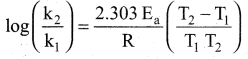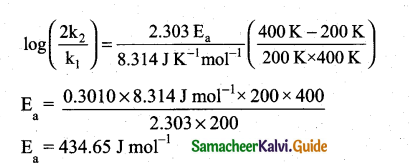Question 8.
The following solutions were prepared by mixing different volumes of NaOH of HC1 different concentrations.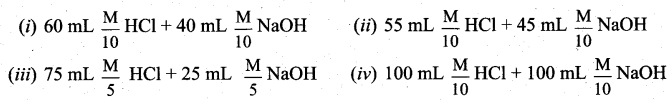pH of which one of them will be equal to 1?
(a) (iv)
(b) (i)
(c) (ii)
(d) (iii)
(d) (iii)
No of moles of HCl = 0.2 × 75 × 10-3 = 15 × 10-3
No of moles of NaOH = 0.2 × 25 × 10-3 = 5 × 10-3
No of moles of HC1 after mixing = 15 × 10-3 – 5 × 10 -3
= 10 × 10-3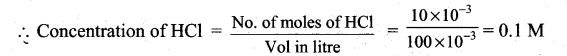for (iii) solution, pH of 0.1MHCl = -log10(0.1) = 1.

Question 9.
The electrode used in SHE is made of ……….
(a) graphite
(b) copper
(c) platinum
(d) iron
(c) platinum

Question 10.
Which one of the following is an example for homogeneous catalysis?
(a) manufacture of ammonia by Haber’s process
(b) manufacture of sulphuric acid by contact process
(c) hydrogenation of oil
(d) Hydrolysis of sucrose in presence of all HCl
Both reactant and catalyst are in same phase. i.e. (1)
(d) Hydrolysis of sucrose in presence of all HCl

Question 11.
The alkaline hydrolysis of fats to give glycerol is known as
(a) Esterification
(b) Hydroboration
(c) Hydration
(d) Saponification
(d) Saponification

Question 12.
Consider the following statements:
(i) In Rosenmund reduction Barium sulphate act as a Catalyst poison palladium catalyst, so that aldehyde cannot be further reduced to alcohol
(ii) Side chain oxidation of toluene using strong oxidising agent gives benzoic acid.
(iii) Friedle crafts reaction is the best method used to prepare aliphatic ketones.
Which of the above statement is/are correct?
(a) (iii) only
(b) (i) & (ii)
(c) (i) & (iii)
(d) (ii) & (iii)
(b) (i) & (ii)Question 13.
Assertion : Acetamide on reaction with KOH and bromine gives acetic acid
Reason : Bromine catalyses hydrolysis of acetamide.
(a) if both assertion and reason are true and reason is the correct explanation of assertion.
(b) if both assertion and reason are true but reason is not the correct explanation of assertion.
(c) assertion is true but reason is false
(d) both assertion and reason are false
(d) both assertion and reason are false

Question 14.
Which is the product formed when fructose undergoes partial reduction with sodium amalgam and water?
(a) Sorbital + mannitol
(b) D-mannose + D-galactose
(c) Gluconic acid + saccharic acid
(d) Aldehyde + ketone
(a) Sorbital + mannitol

Question 15.
The polymer used in making blankets (artificial wool) is
(a) polystyrene
(b) PAN
(c) polyester
(d) polythene
(b) PAN

Part – II

Answer any six questions. Question No. 22 is compulsory. [6 × 2 = 12]

Question 16.
Predict the conditions under which
(а) Aluminium might be expected to reduce magnesia.
(b) Magnesium could reduce alumina.
The conditions under which:
(a) Ellingham diagram is used to predict thermodynamic feasibility of reduction of oxides of one metal by another metal. Any metal can reduce the oxides of other metals that are located above it in the Ellingham diagram. In the Ellingham diagram, for the formation of magnesia (magnesium oxide) occupy lower position than aluminium oxide. Therefore aluminium cannot be used to reduce the oxides of magnesium (magnesia). Above 1623K, A1 can reduce MgO to Mg, so that ArG° becomes negative and the process becomes thermodynamically feasible.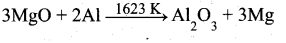(b)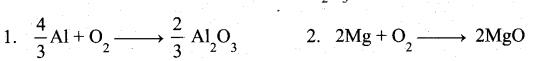At the point of intersection of the Al2O3 and MgO curves in
Ellingham diagram. ∆G0 becomes zero for the reaction.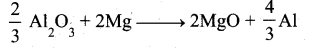Question 17.
Give the uses of carbon dioxide.

• Carbon dioxide is used to produce an inert atomosphere for chemical processing.
• Biologically, it is important for photosynthesis.
• It is also used as fire extinguisher and as a propellent gas.
• It is used in the production of carbonated beverages and in the production of foam.

Question 18.
What is the coordination entity formed when excess of liquid ammonia is added to an aqueous solution copper sulphate?
When excess of liquid ammonia is added to an aqueous solution of copper sulphate to give tetraamminecopper (II) sulphate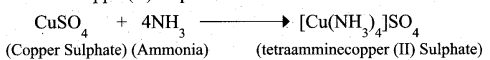Therefore, the coordination entity is [Cu(NH3)4]2+

Question 19.
Atoms X and Y form bcc crystalline structure. Atom X is present at the corners of the cube and Y is at the centre of the cube. What is the formula of the compound?
Atoms X and Y form bcc crystalline structure. Atom X is present at the comers of the cube Atom Y is present at die centre of the cube.
No of atoms of X in the unit cell = $$\frac{N_{c}}{8}=\frac{8}{8}=1$$
No of atoms of Y in the’unit cell = $$\frac{N_{b}}{1}=\frac{1}{1}=1$$
Ratio of atoms X : Y = 1 : 1 .
Hence formula of the compound = XY.

Question 20.
Define zero order reaction. Give the unit for its rate constant(k).
Zero Order Reaction. The reaction in which die rate of reaction is independent of the concentration of the reactants is called zero order reaction.
Rate = k [A]0
= k
Where k is the rate constant. Its unit is mol L-1 s-1

Question 21.
Distinguish between galvanic cell and electrolytic cell.
Galvanic Cell:

1. It is a device in which a spontaneous chemical reaction generates an electric current.
2. It converts chemical energy into electrical energy. It is commonly known as Battery.
3. e.g., Daniel cell, Dry cell.
4. A salt bridge is used in this.

Electrolytic cell :

1. It is a device in which an electric current from an external source drives a non spontaneous reaction
2. It converts electrical energy into chemical energy.
3. e.g., Electrolysis of molten NaCl.
4. Na salt bridge is used.

Question 22.
When phenol is treated with propan-2-ol in the presence of HF, Friedel-Craft reaction takes place. Identify the products.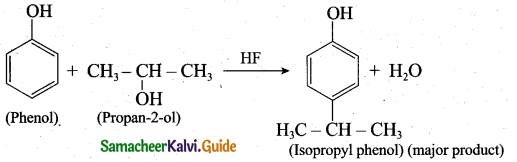Question 23.
Human cannot use cellulose as food – Why?
Human cannot use cellulose as food because our digestive systems do not contain the necessary enzymes such as glycosidases (or) cellulases that can hydrolyse the cellulose. But animals contain cellulose enzyme in their digestive system and they can digest cellulose. So cellulose can used as food for animals but not for human.

Question 24.
What are antibiotics?
Antibiotics is a chemical substance produced by one microorganism, that selectively inhibits die growth of another micro organism. Example : penicillins and cephalosporins.

Part – III

Answer any six questions. Question No. 31 is compulsory. [6 × 3 = 18]

Question 25.
Explain the types of silicones.
(i) Linear silicones: They are obtained by the hydrolysis and subsequent condensation of dialkyl or diaryl silicon chlorides.

• Silicone rubbers: These silicones are bridged together by methylene or similar groups.
• Silicone resins: They are obtained by blending silicones with organic resins such as acrylic esters.

(ii) Cyclic silicones: These are obtained by the hydrolysis of R2SiCl2
(iii) Cross linked silicones: They are obtained by hydrolysis of RSiCl3

Question 26.
Give the properties of inter halogen compounds.
Properties of inter halogen compounds:

• The central atom will be the larger one.
• It can be formed only between two halogen and not more than two halogens.
• Fluorine can’t act as a central metal atom being the smallest one.
• Due to high electronegativity with small size fluorine helps the central atom to attain high coordination number
• They can undergo the auto ionization.
• They are strong oxidizing agents.

Question 27.
Based on VB theory explain why [Cr(NH3 )6]3+ is paramagnetic, while [Ni(CN4)]2- is diamagnetic.
(a) [Cr(NH3 )6]3+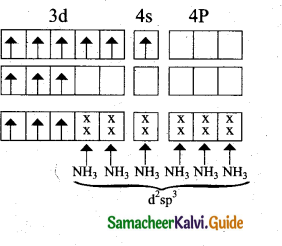In this complex Cr is in the +3 oxidation state.
Electronic configuration of Cr atom
Electronic configuration of Cr+3 ion
Hybridisation and formation of [Cr(NH3)6]+3 Complex

• Due to the presence of three unpaired electrons in [Cr(NH3 )6 ]3+ it behaves as a paramagnetic substance.
• The spin magnetic moment,
$$\mu s=\sqrt{3(3+2)}=\sqrt{15}=3.87 \mathrm{BM}$$
• [Cr(NH3)6]3+ is an inner orbital octahedral complex.

(b) [Ni(CN)4]-2
In this complex Ni is in the +2 oxidation state.
Electronic configuration of Ni atom
Electronic configuration of Ni2+ ion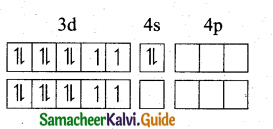Hybridisation and formation of [Ni(CN)4]-2 Complex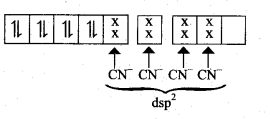Since CN is strong field ligand, hence the electrons in 3d orbitals are forced to pair up and there is no impaired electron in [Ni(CN)4]2-, hence it’should be diamagnetic substance.

Question 28.
A first order reaction is 20% completed in 10 minutes. Calculate the time taken for the reaction to go to 80% completion.
Applying the first order equation,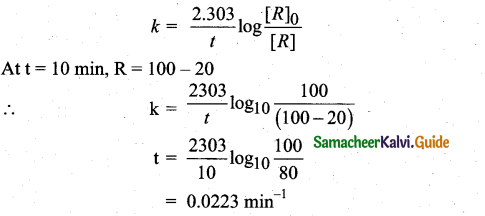Question 29.
Explain common ion effect with an example.
Common Ion Effect: When a salt of a weak acid is added to the acid itself, the dissociation of the weak acid is suppressed further.
Acetic acid is a weak acid. It is not completely dissociated in aqueous solution aid hence the following equilibrium exists.
CH3COOH (aq) ⇌ H+ (aq)+ CH3OO(aq)
However, the added salt, sodium acetate, completely dissociates to produce Na+ and CH3COO ion.
CH3COONa (aq) → Na+(aq) + CH3COO (aq) .

Hence, the overall concentration of CH3COO is increased, and the acid dissociation equilibrium is disturbed. We know from Le chatelier’s principle that when a stress is applied to a system at equilibrium, the system adjusts itself to nullify the effect produced by that stress. So, in order to maintain the equilibrium, the excess CH3COO ions combines with H+ ions to produce much more unionized CH3COOH i.e., the equilibrium will shift towards the left. In other words, the dissociation of CH3COOH is suppressed. Thus, the dissociation of a weak acid (CH3COOH) is suppressed in the presence of a salt (CH3COONa) containing an ion common to the weak electrolyte. It is called the common ion effect.

Question 30.
Mention the uses of Brownian movement.

• Brownian movement enables us to calculate Avogadro Number.
• It is used to confirm kinetic theory which considers the ceaseles rapid movement of molecules that increases with increase in temperature.
• It is used to understand the stability of colloids. As the particles are in continuous rapid movement, they do not come close and hence not get condensed. That is Brownian movement does not allow the particles to be acted on by force of gravity.

Question 31.
How will you convert benzaldehyde into the following compounds?
(i) benzophenone
(ii) benzoic acid
(iii)2 – hydroxyphenylaceticacid.
(i) conversion of benzaldehyde into benzophenone.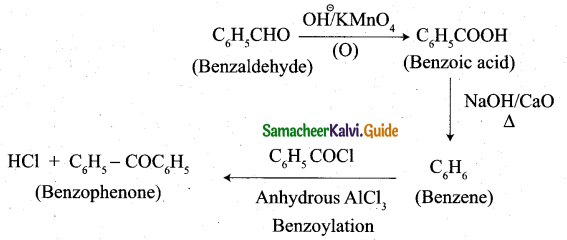(ii) conversion of benzaldehyde into benzoic acid: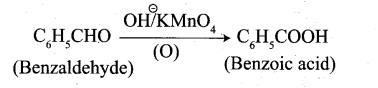(iii) conversion of benzaldehyde into 2 – hydroxy phenyl acetic acid: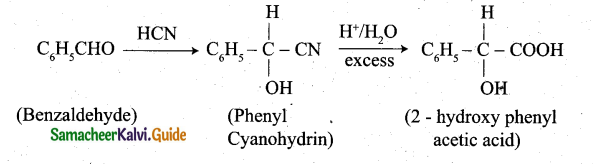Question 32.
Complete the following.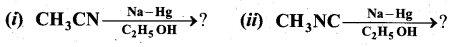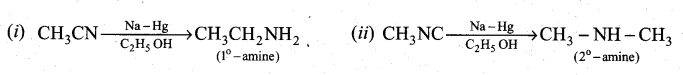Question 33.
(i) What are reducing sugars?
(ii) What is meant by denaturation of a protein?
(iii) How is oxygen replenished in our atmosphere?
(i) Reducing sugar: All those Carbohydrates which reduce Fehling’s solution and Tollens’ reagent are referred to as reducing sugars. All monosaccharides whether aldose or ketose are reducing sugars.

(ii) Denaturation of a protein: When 2° and 3° structure of a protein is destroyed due to the physical changes like temperature, change in pH, it is called denaturation of a protein. Example: Coagulation of egg white on boiling.

(iii) We take oxygen from atmosphere and release CO2. Plants take up CO2 and H2O from the atmosphere to prepare their food in the presence of sunlight and release O2, thus O2 is replenished in atmosphere.Part – IV

Answer all the questions. [5 × 5 = 25]

Question 34.
(a) (i) What is Cementation? (2)
(ii) Write a notes on ionisation enthalpy in p-block elements? (3)
[OR]
(b) (i) Give the uses of sulphuric acid.. (2)
(ii) How alloys are formed in d-block elements? (3)
(а) (i) Gold can be recovered by reacting the deoxygenated leached solution with zinc. In this process the gold is reduced to its elemental state (zero oxidation sate) and the process is
called cementation.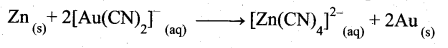(ii) 1. As we move down a group, generally there is a steady decrease in ionisation enthalpy of elements due to increase in their atomic radius.

2. In p-block elements there are some minor deviations to this general trend. In group 13, from B to A1 the ionisation enthalpy decreases as expected. But from A1 to T1 there is only a marginal difference. This is due to the presence of inner d- and f – elements which has poor shielding effect compared to s andp electrons. As a result, the effective nuclear charge on the valance electrons increase.

3. A similar trend is also observed in group 14. The remaining groups (15-18) follows the general trend, in these groups the ionisation enthalpy decreases as we move down the group. Here poor shielding effect of d- and/electrons are overcome by the increased shielding effect of the additional p-electrons.

4. The ionisation enthalpy of elements in successive groups is higher than the corresponding elements of the previous group as expected.

[OR]

(b) (i)

1. Sulphuric acid is used in the manufacture of fertilisers, ammonium sulphate and
super phosphates and other chemicals such as hydrochloric acid, nitric acid etc.
2. It is used as a drying agent and also used in the preparation of pigments, explosives etc.

(ii)

1. An alloy is formed by blending a metal with one or more other elements. The elements may be metals or non-metals or both.
2. The bulk metal is named as solvent, mid the other elements in smaller portion is called solute.
3. According to Hume-Rothery rule to form an alloy, the difference between the atomic radii ofthe solvent and solute is less than 15%. Both the solvent and solute must have the same crystal structure and valence and their electro negativity difference must be close to zero.
4. Since their atomic sizes are similar and one metal atom can be easily replaced by another metal atom from its crystal lattice to form an alloy. The alloys are hard and
have high melting points. Examples: Gold – copper alloy.

Question 35.
(a) (i) A solution of [CO(NH3) 4I2]Cl when treated with AgNO3, gives a white precipitate . What should be the formula of isomer of the dissolved complex that gives yellow precipitate with AgNO3 What are the above isomers called? (2)
(ii) Write the following in the complex [Cr (en)3 [Cr F6]
(i) Type of complex
(ii) Ligands
(iii) central metal
(rv) Oxidation state of central metal
(v) IUPAC name (3)
[OR]
(b) (i) KF crystallizes in fee structure like sodium chloride, calculate the distance between K+ and F in KF. (given : density of KF is 2.48 g cm-3) (3)
(ii) How do concentrations of the reactant influence the rate of reaction? (2)
(a) (i)1. A solution of [CO(NH3)4I2]Cl when treated with AgNO3 gives a white precipitate, because Cl ion is counter ion.
2. Formula of isomer of the dissolved complex that gives yellow precipitate with AgNO3 is, [CO (NH3)4 Cl I] Iθ because Ie is counter ion
3. [CO(NH3)4I2]Cl and [CO(NH3)4 Cl I]I both are ionisation isomers.

(ii)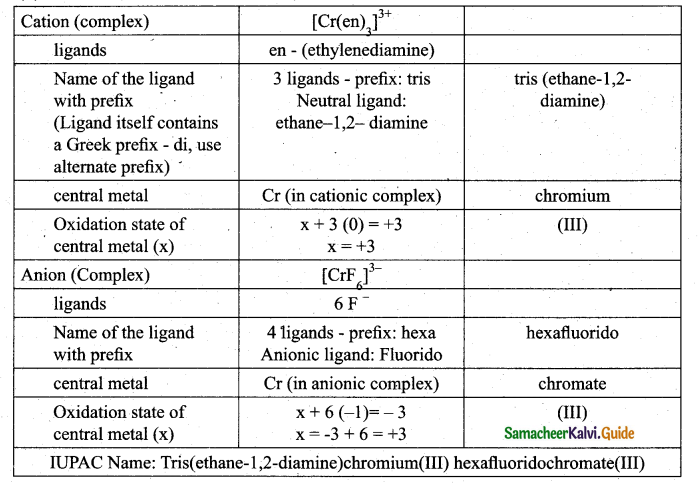[OR]

(b) Density of KF = 2.48cm-3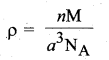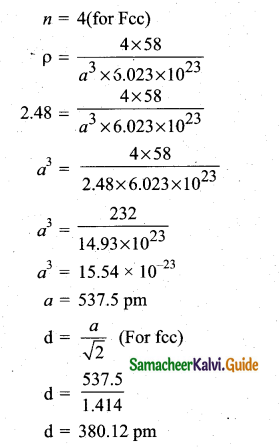(ii) The rate of a reaction increases with the increase in the concentration of the reactants. The effect of concentration is explained on the basis of collision theory of reaction rates. According to this theory, the rate of a reaction depends upon the number of collisions between the reacting molecules. Higher the concentration, greater is the possibility for collision and hence the rate.

Question 36.
(a) (i) Point out the differences between ionic product and solubility product. (2)
(ii) The solubility of AgCl in water at 298 K is 1.06 × 10-5 mole per litre. Calculate is solubility product at this temperature. (3)
[OR]
(b) (i) Describe about lithium-ion battery and its uses. (3)
(ii) Give reasons for the following (2)
(1) Rusting of iron is quicker in saline water than in ordinary water.
(2) Aluminium metal cannot be produced by the electrolysis of aqueous solution of aluminium salt.
(a) (i)

Ionic product

1. It is applicable to all types of solutions.
2. Its value changes with the change in con-centration of the ions.

Solubility product :

1. It is applicable to the saturated solutions.
2. It has a definite value for an electrolyte at a constant temperature.

(ii) The solubility equilibrium in the saturated solution is
AgCl (s) ⇌ Ag+(aq) + Cl (aq)
The solubility of AgCl is 1.06 × 10-5 mole per litre.
[Ag+(aq)] = 1.06 × 10-5molL-1
[Cl (aq)] = 1.06 × 10-5 mol L-1
Ksp = [Ag+(aq)] [Cl(aq)]
= (1.06 × 10-5 mol L-1) × (1.06 × 10-5 mol L-1)
= 1.12 × 10-2 mol2 L-2

[OR]

(b) (i) 1. Lithium-ion battery
Anode : Porous graphite
Cathode : Transition metal oxide as CoO2
Electrolyte : Lithium salt in an organic solvent

2. At the anode oxidation occurs
Li(s) —Li+(aq) + e
At the cathode reduction occurs.
Li+ + CoO2(s) + e → Li CoO2(s)

3. Overall reactions
Li(s) + CoO2 → Li CoO2(s)

4. Both electrodes allow Li+ ions to move in and out of their structures. During discharge the Li+ ions produced at the anode moves towards cathode through the non-aquaeous electrolyte.

5. When a potential greater than the emf produced by the cell is applied across the electrode, the cell reaction is reversed and now the Li+ ions move from cathode to anode where they become embedded on the porous electrode. This is known as intercalation.
6. Uses:- This Li-ion battery is used in cellular phones, Laptop computer and digital camera.

(ii)

1. It is because in saline water, there are more H+ ions. Greater the number of H+ ions, quicker the rusting will take place.
2. It is because aluminium metal is more reactive than hydrogen aid it will react with H2O.Question 37.
(a) (i) Heat of adsorption is greater for chemisorptions than physisorption. Why? (2)
(ii) Give three examples for heterogeneous catalysis. (3)
[OR]
(b) (i) How is Aniline converted into Phenol? (2)
(ii) What is the action of HCN on
(1) propanone (2) 2,4-dichlorobenzaldehyde. (3)
(a) (i) Chemisorption has higher heat of adsorption, because in chemisorption the chemical bonds are much stronger. In adsorbed state the adsorbate is hold on the surface of adsorbent by attractive forces (bond). And chemisorption is irreversible one. Therefore, heat of adsorption is greater for chemisorptions than physisorption.
Chemisorption, heat of adsorption range 40-400kJ/mole.

(ii)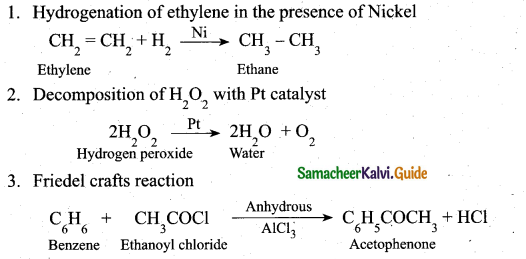[OR]

(b) (i) Aniline is diazotized with nitrous acid (NaN02 + HC1) at 273-278K to give benzene diazonium chloride which on further treatment with hot water in the presence of mineral acid gives phenol.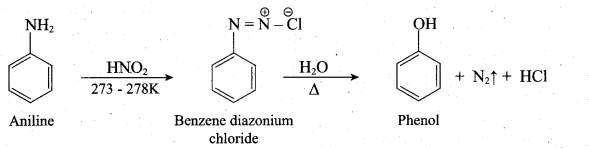(ii) 1. Propanone reacts with HCN: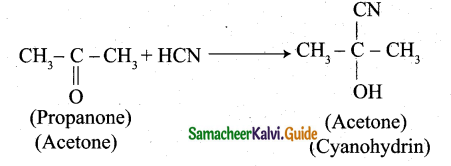2. 2,4 – dichlorobenzaldehyde reacts with HCN: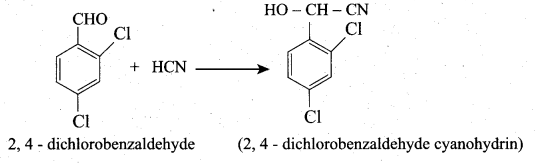Question 38.
(a) (i) An aromatic compound ‘A’ of molecular formula C7H7ON undergoes a series of reactions as shown below. Write the structures of A, B, C, D and E in the following reactions. (5)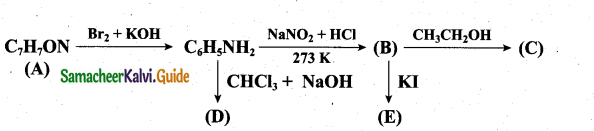[OR]

(b) (i) Write a note on co -polymer. (3)
(ii) What is the difference between elastomers and fibres? Give one example of each.(2)
a(i)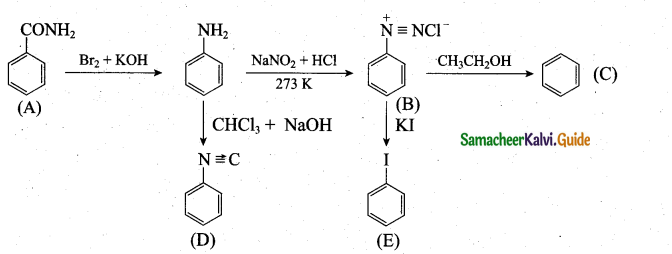[OR]

(b) (i)

1. A polymer containing two or more different kinds of monomer units is called a copolymer.
2. Co-polymers have properties quite different from the homopolymers.
3. The structural units of co-polymers are derived from the different monomers may be present in regular, alternation or in random order or strings of several units of one kind may alternate with strings of another.
4.  For example, Buna – S, Buna – N, Nylon – 6,6 etc.
Buna – S contains styrene and butadiene monomer units.

Elastomers :

1. These are rubber like solids with elastic properties.
2. These are held by the weak inter- molecular forces.
Example: Buna-S and Buna-N.

Fibres :

1. These are the thread forming solids which possess high tensile strength and high modulus.
These are held together by strong intermolecular forces like hydrogen bonding. Example: Nylon 6, 6 and
2. polyesters (terylene)